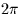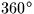Next: SLA_CR2TF - Radians to Hour,Min,Sec
Up: SUBPROGRAM SPECIFICATIONS
Previous: SLA_COMBN - Next Combination

## SLA_CR2AF - Radians to Deg,Arcmin,Arcsec

ACTION:
Convert an angle in radians to degrees, arcminutes, arcseconds (single precision).

CALL:
CALL sla_CR2AF (NDP, ANGLE, SIGN, IDMSF)

GIVEN:

 I NDP number of decimal places of arcseconds ANGLE R angle in radians

RETURNED:

 C SIGN +' or -' IDMSF I(4) degrees, arcminutes, arcseconds, fraction

NOTES:
1.
NDP less than zero is interpreted as zero.
2.
The largest useful value for NDP is determined by the size of ANGLE, the format of REAL floating-point numbers on the target machine, and the risk of overflowing IDMSF(4). For example, on a VAX computer, for ANGLE up to, the available floating-point precision corresponds roughly to NDP=3. This is well below the ultimate limit of NDP=9 set by the capacity of the 32-bit integer IHMSF(4).
3.
The absolute value of ANGLE may exceed. In cases where it does not, it is up to the caller to test for and handle the case where ANGLE is very nearlyand rounds up to, by testing for IDMSF(1)=360 and setting IDMSF(1-4) to zero.Next: SLA_CR2TF - Radians to Hour,Min,Sec
Up: SUBPROGRAM SPECIFICATIONS
Previous: SLA_COMBN - Next Combination

SLALIB --- Positional Astronomy Library